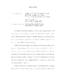## Adaptive Finite Element Methods for Variational Inequalities: Theory and Applications in Finance

 dc.contributor.advisor Nochetto, Ricardo H en_US dc.contributor.author Zhang, Chensong en_US dc.contributor.department Applied Mathematics and Scientific Computation en_US dc.contributor.publisher Digital Repository at the University of Maryland en_US dc.contributor.publisher University of Maryland (College Park, Md.) en_US dc.date.accessioned 2007-09-28T14:56:38Z dc.date.available 2007-09-28T14:56:38Z dc.date.issued 2007-05-29 en_US dc.description.abstract We consider variational inequalities (VIs) in a bounded open domain Omega subset IR^d with a piecewise smooth obstacle constraint. To solve VIs, we formulate a fully-discrete adaptive algorithm by using the backward Euler method for time discretization and the continuous piecewise linear finite element method for space discretization. The outline of this thesis is the following. Firstly, we introduce the elliptic and parabolic variational inequalities in Hilbert spaces and briefly review general existence and uniqueness results. Then we focus on a simple but important example of VI, namely the obstacle problem. One interesting application of the obstacle problem is the American-type option pricing problem in finance. We review the classical model as well as some recent advances in option pricing. These models result in VIs with integro-differential operators. Secondly, we introduce two classical numerical methods in scientific computing: the finite element method for elliptic partial differential equations (PDEs) and the Euler method for ordinary different equations (ODEs). Then we combine these two methods to formulate a fully-discrete numerical scheme for VIs. With mild regularity assumptions, we prove optimal a priori convergence rate with respect to regularity of the solution for the proposed numerical method. Thirdly, we derive an a posteriori error estimator and show its reliability and efficiency. The error estimator is localized in the sense that the size of the elliptic residual is only relevant in the approximate noncontact region, and the approximability of the obstacle is only relevant in the approximate contact region. Based on this new a posteriori error estimator, we design a time-space adaptive algorithm and multigrid solvers for the resulting discrete problems. In the end, numerical results for $d=1,2$ show that the error estimator decays with the same rate as the actual error when the space meshsize and the time step tend to zero. Also, the error indicators capture the correct local behavior of the errors in both the contact and noncontact regions. en_US dc.format.extent 2180519 bytes dc.format.mimetype application/pdf dc.identifier.uri http://hdl.handle.net/1903/7167 dc.language.iso en_US dc.subject.pqcontrolled Mathematics en_US dc.subject.pqcontrolled Mathematics en_US dc.subject.pquncontrolled finite element method en_US dc.subject.pquncontrolled adaptive algorithm en_US dc.subject.pquncontrolled variational inequality en_US dc.subject.pquncontrolled option pricing en_US dc.subject.pquncontrolled multigrid en_US dc.subject.pquncontrolled parabolic en_US dc.title Adaptive Finite Element Methods for Variational Inequalities: Theory and Applications in Finance en_US dc.type Dissertation en_US
##### Original bundle
Now showing 1 - 1 of 1